First AKD2G Tuning | 29 Aug 2022 | |

# First AKD2G Tuning

Users facing the tuning of an AKD2G servodrive for the first time will have to use the Workbench configuration software tool which, for novice users or users of the Servostar series drive, may confuse them given the difference in the graphical interface and the many features introduced. This note will guide you in setting a general configuration indicating the most significant parameters to be configured.

### Tuning Mode

Workbench allows different tuning modes. The more experienced user will appreciate Performance Servo Tuner while the less experienced user can easily use the Slider Tuning.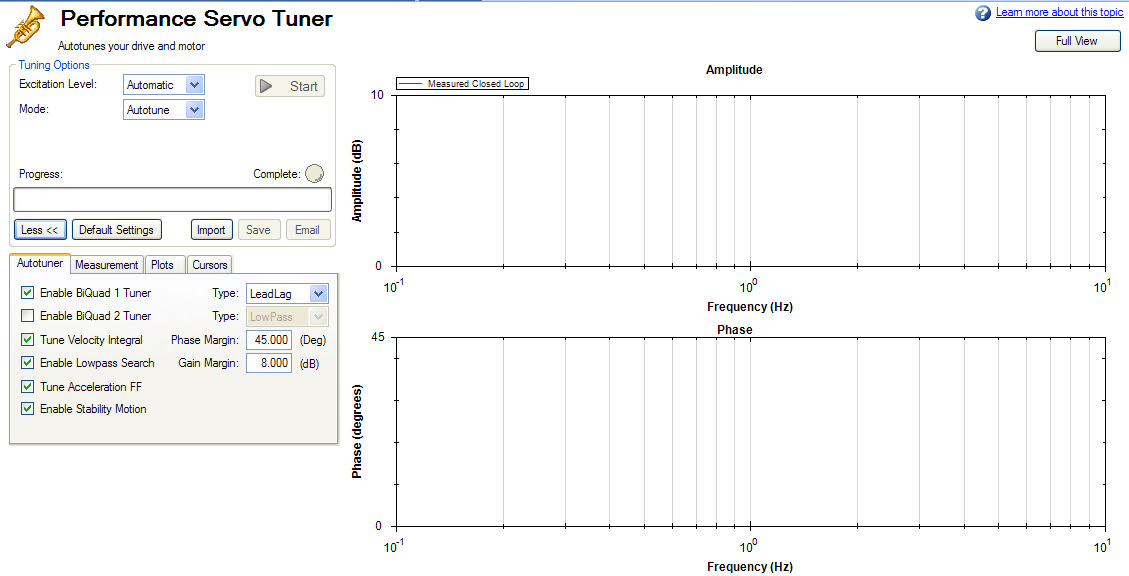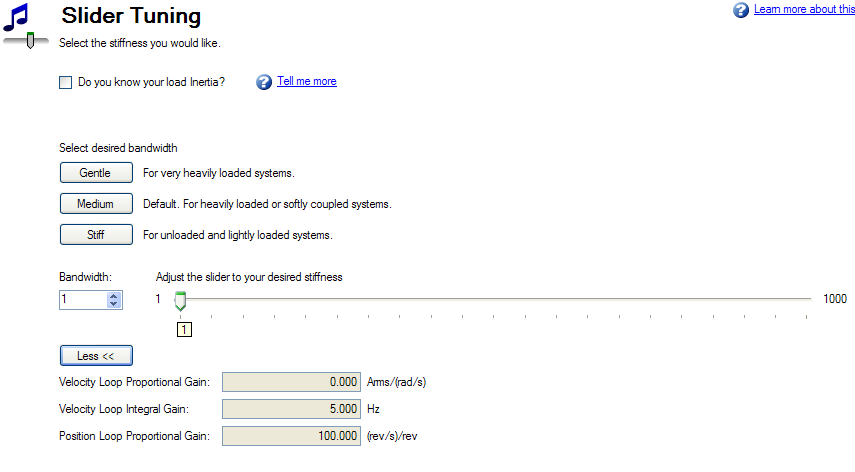However, both the first case and the second one may encounter difficulties linked to the mechanical and kinematic characteristics of the load to be handled (vertical load, limited stroke, resonance frequencies, protection intervention, etc.). All this can make it complicated if not impossible to perform an automatic tuning.
Given the large number of parameters of the AKD2G, only the parameters necessary for the regulation will be illustrated and set below to obtain a first reliable operation applicable to any mechanical and kinematic situation.
For the moment we leave out the Performance Servo Tuner and Slider Tuning.
It is possible that the suggested values will have to be modified later, both to obtain a fine-tuning of the kinematics and in case of kinematic problems.

### Manual Tuning

The parameters set below refer to the speed and position loop. The user can adopt the suggestions by acting on the parameters shown in the screens of the windows involved in the various functions or manually using the terminal function.

 Velocity Loop PI Controller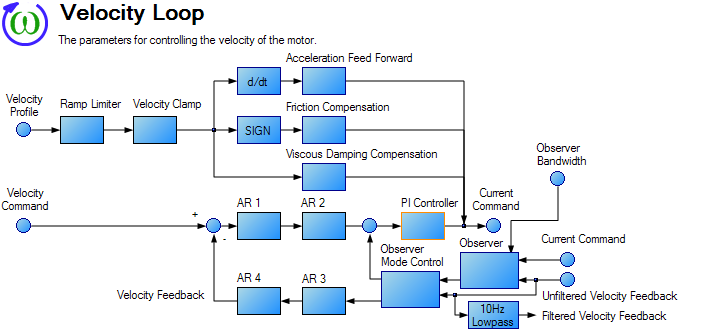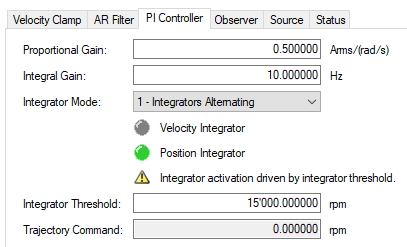The integral gain will initially be set to 10 Hz.

AXIS1.VL.KI = 10

The proportional gain value can initially be calculated with this formula:

AXIS1.VL.KP = 500 * Jtot / Kt

where:

Jtot = load inertia + motor inertia (Kgm^2)
Kt = motor torque constant (Nm/A)

If the inertia of the load compared to the motor shaft is not known, set a value from those in the table present according to the rated power of the motor power Pn:

 Pn < 1kW VL.KP=0.02 1kW < Pn < 2kW VL.KP=0.08 2kW < Pn < 8kW VL.KP=1 Pn > 8kW VL.KP=4

Starting from these initial values, increase AXIS1.VL.KP until the motor starts to vibrate, then reduce it.

#### AR Filter

If the motor has high resolution feedback (optical sin-cos encoder, Hiperface, EnDat 2.1 oe 2.2 full digital) set up filters in this way:

 AR2,AR3 e AR4 = Unity Gain AR1 = BiQuad

In summary:

 AXIS1.VL.ARTYPE1 = 4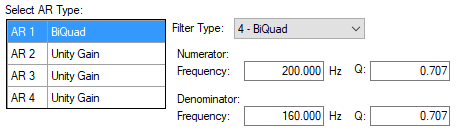AXIS1.VL.ARTYPE2 = 0 AXIS1.VL.ARTYPE3 = 0 AXIS1.VL.ARTYPE4 = 0 AXIS1.VL.ARZF1 = 200 AXIS1.VL.ARPF1 = 160 AXIS1.VL.ARPQ1 = 0.707 AXIS1.VL.ARZQ1 = 0.707

If the motor has low resolution feedback (TTL square wave encoder, resolver, SFD3) of Hiperface DSL encoder set up filters in this way:

AR2 = Unity Gain
AR1, AR3 e AR4 = BiQuad

In summary:

 AXIS1.VL.ARTYPE1 = 4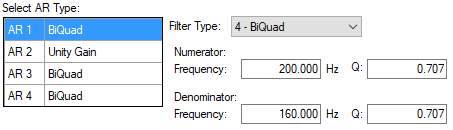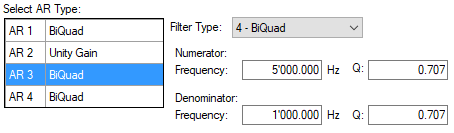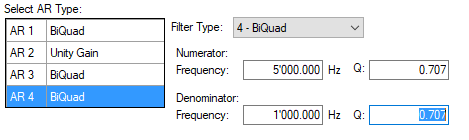AXIS1.VL.ARTYPE2 = 0 AXIS1.VL.ARTYPE3 = 4 AXIS1.VL.ARTYPE4 = 4 AXIS1.VL.ARZF1 = 200 AXIS1.VL.ARPF1 = 160 AXIS1.VL.ARPQ1 = 0.707 AXIS1.VL.ARZQ1 = 0.707 AXIS1.VL.ARZF3 = 5000 AXIS1.VL.ARPF3 = 1000 AXIS1.VL.ARPQ3 = 0.707 AXIS1.VL.ARZQ3 = 0.707 AXIS1.VL.ARZF4 = 5000 AXIS1.VL.ARPF4 = 1000 AXIS1.VL.ARPQ4 = 0.707 AXIS1.VL.ARZQ4 = 0.707

#### Observer (to be used mainly with low-resolution feedback)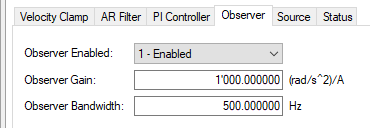Observer Mode AXIS1.OBS.ENABLE = 1 Observer gain AXIS1.OBS.KO = Kt/Jtot (motor torque constant divided by total inertia) Observer Bandwidth AXIS1.OBS.BW = 500

If you do not know the inertia of the load, use the inertia of the motor multiplied by 2 for the calculation:

Jtot=Jm*2

#### Position Loop

Skip this part if the AKD operating mode is different from Position Mode.

#####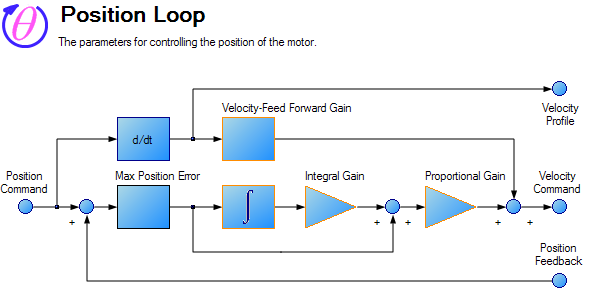Gains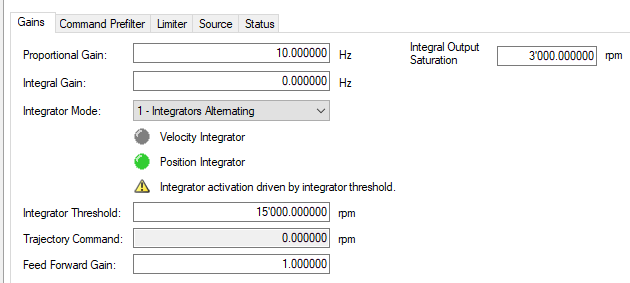Set the initial parameters like this:

 AXIS1.PL.KP = 10 AXIS1.PL.KI = 0 AXIS1.VL.KVFF = 1

#### Limits

Set the limits as follows:

 AXIS1.IL.LIMITP = x (application requirements in A) AXIS1.IL.LIMITN = -x (application requirements in A) AXIS1.VL.VFTHRESH = y (application requirements, e.g. motor max speed multiplied by 1.2)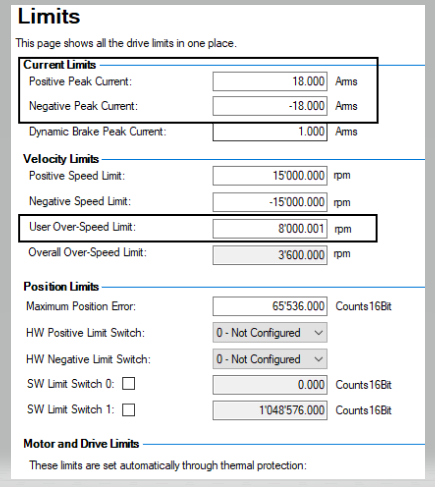### Further Considerations

It should be clear that the values of the speed gains (AXIS1.VL.KP and AXIS1.VL.KI) and of the position (AXIS1.PL.KP) will be optimized according to the result to be achieved. AXIS1.VL.KP is proportional to the total inertia so it can be increased in steps until reaching a high motor noise, and then decrease it to the value of the previous step. AXIS1.PL.KP can instead be increased until the desired tracking error is reached, considering that a too high value may cause overshoot of speed or oscillations at low speed. Finally, AXIS1.VL.KI must be commensurate with the overall inertia of the system (lower than 10Hz for high inertia, higher but lower than 50Hz for low inertia).